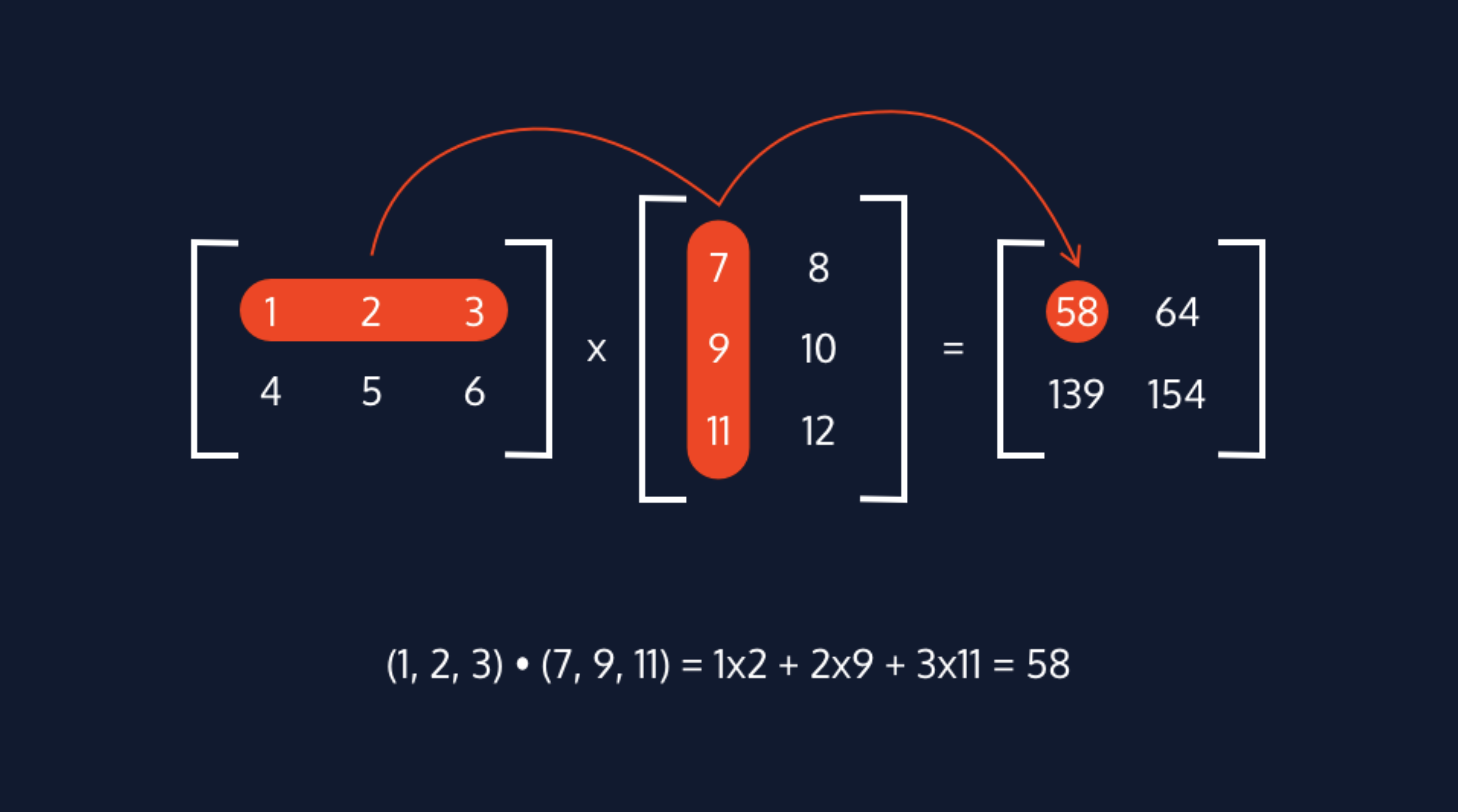# Introduction to Deep Learning

Print Cheatsheet

### Scalars, Vectors, and Matrices

Scalars, vectors, and matrices are fundamental structures of linear algebra, and understanding them is integral to unlock the concepts of deep learning.

• A scalar is a singular quantity like a number.
• A vector is an array of numbers (scalar values).
• A matrix is a grid of information with rows and columns.

They can all be represented in Python using the NumPy library.

import numpy

# Scalar code example
x = 5

# Vector code example
x = numpy.array([1, 2, 3, 4])

# Matrix code example
x = numpy.array([1, 2, 3], [4, 5, 6], [7, 8, 9])

### Tensors

A tensor is a multidimensional array and is a generalized version of a vector and matrix. It is the fundamental data structure used in deep learning models.

import numpy as np

# A 0-D tensor would be a scalar
x = 5

# A 1-D tensor would be a vector
x = numpy.array([1, 2, 3])

#A 2-D tensor would be a matrix
x = numpy.array([[1, 2], [3, 4], [5, 6]])

# A 3-D tensor would be a "cube" or a "stack" of matrices
x = numpy.array([[[1, 2, 3], [4, 5, 6]], [[7, 8, 9], [10, 11, 12]]])

### Neural Network Concept Overview

Neural networks are made up of multiple layers of computational units called nodes. The different types of layers an input layer can have are:

• Input Layer: The layer where inputs enter the neural network. There are as many nodes in the input layer as there are features in the input data point.
• Hidden layer: A layer that comes between the input layer and the output layer. They introduce complexity into a neural network and help with the learning process. One can have as many hidden layers as you want in a neural network (including zero).
• Output Layer: The final layer in our neural network. It produces the final result, so every neural network must have only one output layer.

These nodes are connected to each other via weights, which are the learning parameters of a deep learning model, determining the strength of each linked node’s connection. The weighted sum between nodes and weights is calculated with the following formula:

$weighted\_sum = (inputs \cdot weight\_transpose) + bias\_node$### Activation Functions and Forward Propagation

Activation functions are applied to the weighted sums between weights and connected nodes, and they determine what is fired to a neuron in the next layer of the neural network. Two common activation functions are ReLU and sigmoid. An example of the sigmoid function is shown in the image.

The bias node shifts the activation function either left or right to create the best fit for the given data.

$Activation(weighted\_sum + bias\_node)$

This process of firing data through each layer of a neural network is called forward propagation.After inputs have gone through a neural network one time, predictions are unlikely to be accurate. Thus, gradient descent (GD) is used to update the weight parameters between neurons to iteratively minimize the loss function and increase the accuracy of the learning model. The process of calculating these gradients is known as backpropagation.

When viewing the GD concept graphically, you will see that it looks for the minimum point of our loss function. Starting at a random point on the loss function, gradient descent will take “steps” in the “downhill direction” towards the negative gradient. The size of the “step” taken is dependent on the learning rate. Choosing an optimal learning rate affects both the accuracy and efficiency of the learning model’s results, as shown in the gif. The formula used with learning rate to update our weight parameters is the following:

\begin{aligned} parameter\_new=parameter\_old+learning\_rate \cdot \\ gradient(loss\_function(parameter\_old)) \\ \end{aligned}### Loss Functions

After data has been sent through a neural network, the error between the predicted values and actual values of the training data is calculated using a loss function. There are two commonly used loss calculation formulas:

• Mean squared error which is used for regression problems — the gif above shows how mean squared error is calculated for a line of best fit in linear regression.
• Cross-entropy loss, which is used for classification learning models rather than regression.Deep learning models are often trained on extremely large datasets. For the sake of efficiency, variations of gradient descent that do not iterate through all data points one at a time are almost exclusively used. The most common ones used are:

• Stochastic Gradient Descent (SGD): A variant of gradient descent where instead of using all data points to update weight parameters, a random data point is selected.
• Adam Optimization: A variant of SGD that allows for adaptive learning rates.
• Mini-batch Gradient Descent: A variant of gradient descent that uses random batches of data to update parameters instead of a random data point.### Scalar Multiplication with Matrices

In scalar multiplication, we multiply every entry of the matrix by the scalar value.### Matrix Multiplication

In matrix multiplication, the number of rows of the first matrix must be equal to the number of columns of the second matrix. The dot product between each row and column of the matrix and placed as an entry into the resulting matrix as shown in the image.### Matrix Transpose

A matrix transpose switches the rows and columns of a matrix.Career Path

### Data Scientist

Beginner friendly

85 Lessons